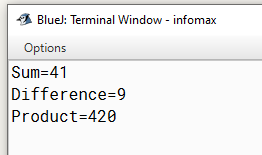# Write a program to find the product of 5, 7 and 12 using variables.

Write a program to find the product of 5, 7 and 12 using variables.

`Solution`

``````class Demo
{
public static void main(String arr[])
{
int a=5,b=7,c=12,d;
d=a*b*c;
System.out.println("Product="+d);
}
}
``````
`Output`